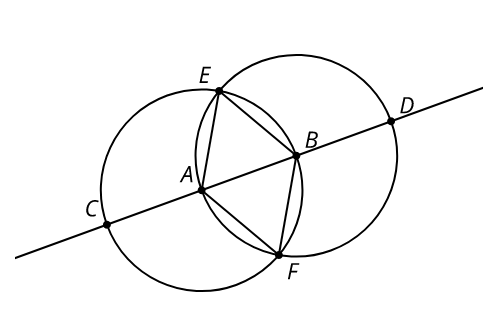# Math Talk: Why Is That True?

Topic:
Geometry
Here are 2 circles with centers andBased on the diagram, explain how you know each statement is true.

## 1. a.

The length of segment is equal to the length of segment .

## 1. b.

Triangle is equilateral.